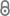# Aggregation in Overlay Networks

K. Hinnenthal, Aggregation in Overlay Networks, Universität Paderborn, 2016.223-MasterarbeitHinnenthal.pdf 1.13 MB
Mastersthesis | English
Supervisor
Department
Abstract
We consider the problem of aggregation in overlay networks. We use a synchronous time model in which each node has polylogarithmic memory and can send at most a polylogarithmic number of messages per round. We investigate how to quickly compute the result of an aggregate functionf over elements that are distributed among the nodes of the network such that the result is eventually known by a selected root node. We show how to compute distributive aggregate functions such as SUM, MAX, and OR in time $O(\log n / \log\log n)$ using a tree that is created in a pre-processing phase. If only a polylogarithmic number of data items need to be aggregated, we show how to compute the result in time $O(\sqrt{\log n / \log\log n})$. Furthermore, we show how to compute holistic aggregate functions such as DISTINCT, SMALLEST(k) and MODE(k) in time $O(\log n / \log\log n)$. Finally, we show a lower bound of $\Omega(\sqrt{\log n / \log\log n})$ for deterministic algorithms that compute any of the aggregate functions in the scope of the thesis.
Publishing Year
LibreCat-ID

### Cite this

Hinnenthal K. Aggregation in Overlay Networks. Universität Paderborn; 2016.
Hinnenthal, K. (2016). Aggregation in Overlay Networks. Universität Paderborn.
@book{Hinnenthal_2016, title={Aggregation in Overlay Networks}, publisher={Universität Paderborn}, author={Hinnenthal, Kristian}, year={2016} }
Hinnenthal, Kristian. Aggregation in Overlay Networks. Universität Paderborn, 2016.
K. Hinnenthal, Aggregation in Overlay Networks. Universität Paderborn, 2016.
Hinnenthal, Kristian. Aggregation in Overlay Networks. Universität Paderborn, 2016.
Main File(s)
File Name
223-MasterarbeitHinnenthal.pdf 1.13 MB
Access LevelClosed Access